## A very quick exercise in L'Hopital's rule

2022 October 10

When some ratio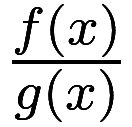has a limit in an “indeterminate form”, in that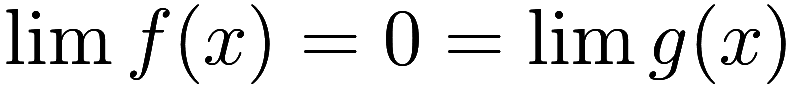, L’Hopital’s rule (first discovered by Bernoulli) allows for calculating the limit: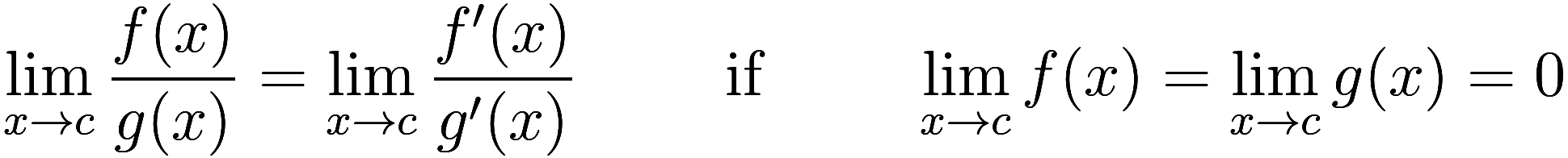under certain conditions. And if at first you don’t succeed, try, try again: when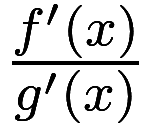is also in indeterminate form, you can differentiate as many times as needed.

As an aside, if we suppose that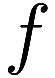and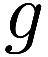can be approximated as Taylor series near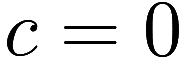, one can easily find that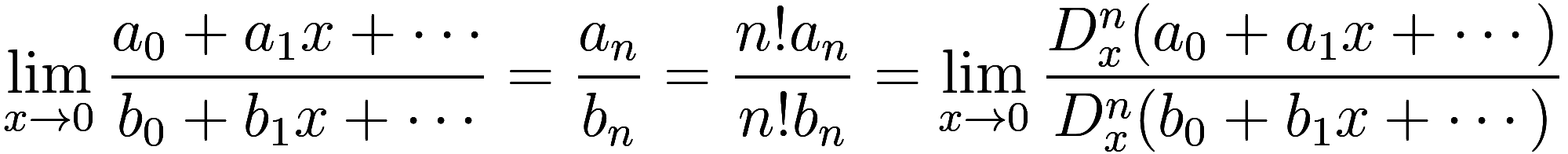when the leading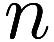terms of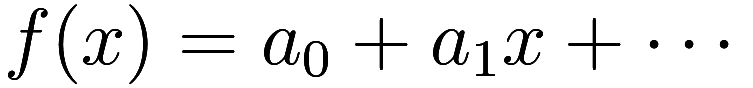and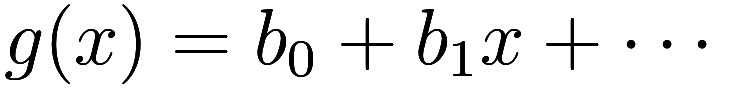are both zero, representing the case wheredifferentiations are needed. Here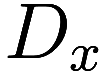represents the differentiation-with-respect-to-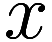operator.

While I have occasionally run into cases where multiple applications of L’Hopital’s rule are needed, the other day1by which I mean 7 years ago… I am a bit slow at updating this blog I was struck by a situation which necessitated a recursive application of L’Hopital’s rule. Consider the Lambert W function which is defined implicitly by the equation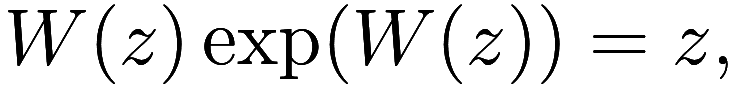that is,is the inverse of the function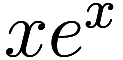. We will only be interested in the principal real branch, which is defined for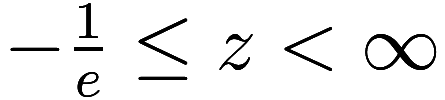. Note that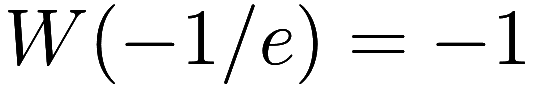. Also, per Wikipedia, we have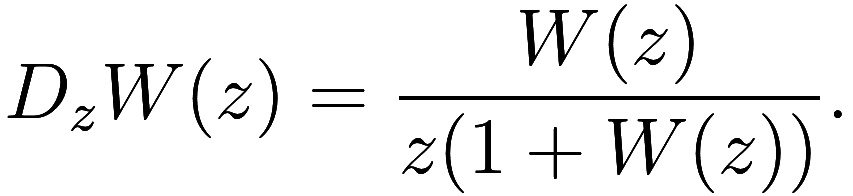Plot of the two real branches of the Lambert W function. The principal branch is labeled .

Ok, now the calculation I ran into in my research was: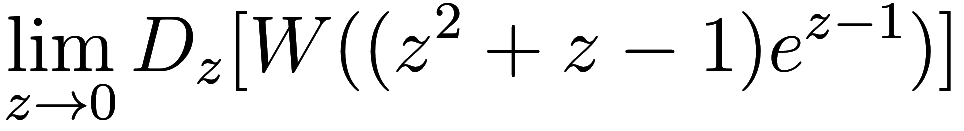Let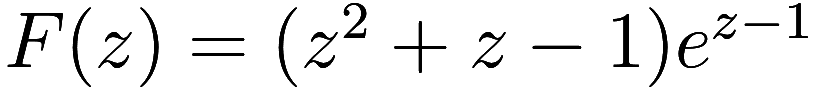and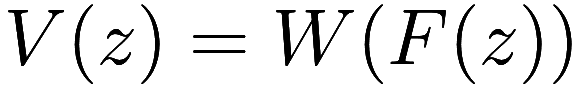so our goal is to calculate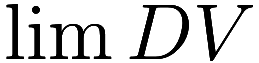where the limit goes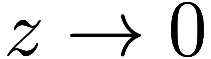.

Note that. Starting by applying the chain rule to the derivative of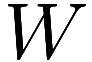, we have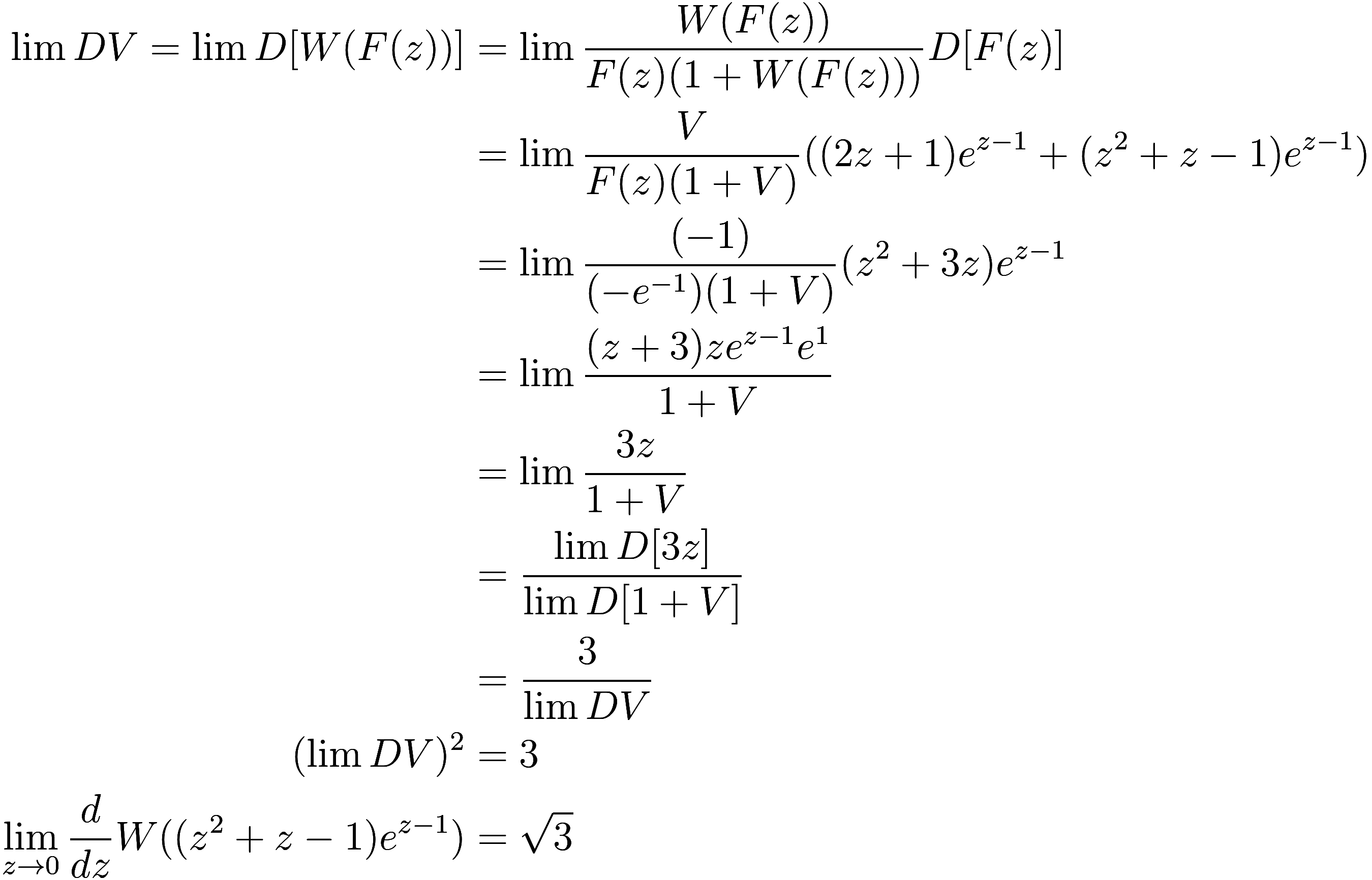From what initially, as I was first calculating it, looked like it would blow up endlessly, instead comes a surprisingly simple and clean result!

Thanks Harry Altman for identifying a mistake in an earlier draft.

1. by which I mean 7 years ago… I am a bit slow at updating this blog↩︎